# High School Physics : Thermodynamics

## Example Questions

← Previous 1 3 4

### Example Question #1 : Thermodynamics

Three substances are added to a mug to make coffee: the coffee, which is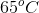, the milk, which is, and the sugar, which is in thermal equilibrium with the coffee. Describe the thermal state of the sugar.

None of these conclusions are valid

The sugar is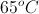, based on the second law of thermodynamics

Heat will be transferred from the sugar to the coffee to reach equilibrium

The sugar is in equilibrium with the milk, based on the zeroth law of thermodynamics

Heat will be transferred from the coffee to the sugar to reach equilibrium

The sugar is in equilibrium with the milk, based on the zeroth law of thermodynamics

Explanation:

The zeroth law of thermodynamics states that if two objects are in thermodynamic equilibrium with a third object, then they must be in thermodynamic equilibrium with each other. In this question, the coffee is in equilibrium with both the milk and the sugar, allowing us to conclude that the milk and sugar must be in equilibrium with each other.

The second law of thermodynamics states that the entropy of the universe is always increasing, and is not relevant to this particular scenario.

### Example Question #2 : Thermodynamics

System A is in equilibrium with system C.

System B is in equilibrium with system C.

System A is in equilibrium with system B according to which law of thermodynamics?

The first law of thermodynamics

The second law of thermodynamics

The universal law of thermodynamics

The third law of thermodynamics

The zeroth law of thermodynamics

The zeroth law of thermodynamics

Explanation:

The zeroth law of thermodynamics states that if two separate systems are in equilibrium with a third system, then they are in equilibrium with each other. The zeroth law of thermodynamics is essentially equivalent to the transitive property of mathematics.

If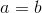and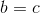, then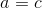.

The first law of thermodynamics states that internal energy changes due to heat flow. Mathematically, this law is presented as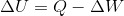.

The second law of thermodynamics states that the entropy (or disorder) of the universe is always increasing. Certain systems exist in which there is a local decrease in entropy, but these processes are always balanced by an increase of entropy outside of the system.

The third law of thermodynamics states that absolute zero is the state in which a system has zero entropy. Essentially, this means that it is impossible to reach absolute zero (at least with modern technology).

### Example Question #3 : Thermodynamics

Gas A is in thermal equilibrium with gases B and C. Which of the following is a valid conclusion?

Thermal equilibrium of gas B is directly proportional to that of gas C

Gases A and B have equal amounts of entropy

No conclusion can be drawn

Thermal equilibrium of gas B is indirectly proportional to that of gas C

Gases B and C are in thermal equilibrium with each other

Gases B and C are in thermal equilibrium with each other

Explanation:

The zeroth law of thermodynamics states that if two systems are in thermal equilibrium with a third system, they are in equilibrium with each other. If gas A is in equilibrium with gas B and gas C, then gas be and gas C must be in thermal equilibrium with each other.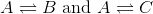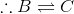### Example Question #4 : Thermodynamics

A gas in a closed container is heated with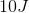of energy, causing the lid of the container to rise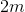with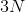of force. What is the total change in energy of the system?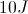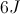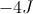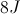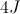Explanation:

For this problem, use the first law of thermodynamics. The change in energy equals the increase in heat energy minus the work done.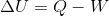We are not given a value for work, but we can solve for it using the force and distance. Work is the product of force and displacement.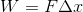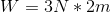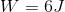Now that we have the value of work done and the value for heat added, we can solve for the total change in energy.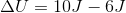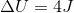### Example Question #1 : Understanding The First Law Of Thermodynamics

A gas in a closed container is heated, causing the lid of the container to rise. The gas performsof work to raise the lid, such that is has a final total energy of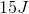. How much heat energy was added to the system?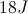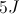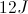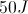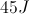Explanation:

For this problem, use the first law of thermodynamics. The change in energy equals the increase in heat energy minus the work done.We are given the amount of work done by the gas and the total energy of the system. Using these values, we can solve for the heat added.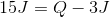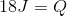### Example Question #6 : Thermodynamics

A gas in a closed container is heated with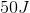of energy, causing the lid of the container to rise. If the change in energy of the system is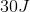, how much work was done by the system?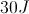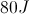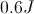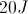Explanation:

For this problem, use the first law of thermodynamics. The change in energy equals the increase in heat energy minus the work done.We are given the total change in energy and the original amount of heat added. Using these values, we can solve for the work done by the system.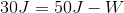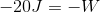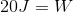### Example Question #7 : Thermodynamics

Which of the following represents the first law of thermodynamics?

If a refrigerator is unplugged, eventually everything inside of it will return to room temperature

While melting, an ice cube remains at the same temperature

Two systems in equilibrium with a third system are in equilibrium with each other

The specific heat of an object explains how easily it changes temperatures

After falling down the hill, a ball's kinetic energy plus heat energy equals the initial potential energy

After falling down the hill, a ball's kinetic energy plus heat energy equals the initial potential energy

Explanation:

The first law of thermodynamics is another wording of the law of conservation of energy. Effectively it states that energy cannot be created or destroyed, but it can change forms.

This means that, in the given situation of the ball rolling down the hill, the total initial energy equals the final kinetic energy plus heat.

The zeroth law of thermodynamics states that if a system is in equilibrium with two other systems, then the two other systems are in equilibrium with each other.

The second law of thermodynamics states that the entropy of a closed system will always increase.

The third law of thermodynamics states that absolute zero is the temperature at which entropy is zero.

### Example Question #8 : Thermodynamics

According to the first law of thermodynamics, in an isothermal process __________.

temperature is not affected by the pressure or volume changes of the system

a constant amount of heat is added to the system

temperature is not affected by the internal energy of the system, work, or heat

it can be implied that heat added to the system is equal to work done by the system

internal energy of the system fluctuates and there exists an imbalance between work and heat

it can be implied that heat added to the system is equal to work done by the system

Explanation:

According to the first law of thermodynamics, change in internal energy of a closed system is given by the difference between the heat energy added to the system and the work done by the system: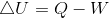In an isothermal process, temperature is constant. Temperature is a measure of internal energy of the system. If temperature is constant, then there is no fluctuation of internal energy.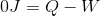It can be implied that heat added to the system is equal to work done by the system.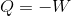### Example Question #1 : Thermodynamics

A glass of cold water is placed in a sealed room. After an infinite amount of time, what will happen?

The water will warm and the air in the room will cool, until they are the same temperature

The water will warm to the temperature of the air

Both the water and the air will remain the same temperature, unless an outside force is applied

The air will cool to the temperature of the water

There is insufficient information to solve

The water will warm and the air in the room will cool, until they are the same temperature

Explanation:

The second law of thermodynamics states that closed systems constantly move towards a state of thermal equilibrium. Since we are looking at a closed system, that means we must be moving towards a state of equilibrium. The only way that happens is if the air cools and the water warms, until they both reach a new final temperature.

Looking at this question in terms of heat transfer, we can infer that the glass of water will warm up, but there must be a transfer of heat to the glass in order for this to occur. The heat comes from the air, causing it to cool as the glass warms.

### Example Question #10 : Thermodynamics

An object can never reach absolute zero in a finite number of steps. Which of these laws supports this statement?

Third law of thermodynamics

Newton's first law

Second law of thermodynamics

First law of thermodynamics

This statement is not supported by a standard law of physics